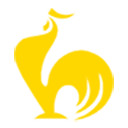ChickenHouse

# 箭头函数，基础知识 #

``````let func = (arg1, arg2, ..., argN) => expression;
``````
1

``````let func = function(arg1, arg2, ..., argN) {
return expression;
};
``````
1
2
3

``````let sum = (a, b) => a + b;

/* 这个箭头函数是下面这个函数的更短的版本：

let sum = function(a, b) {
return a + b;
};
*/

alert( sum(1, 2) ); // 3
``````
1
2
3
4
5
6
7
8
9
10

• 如果我们只有一个参数，还可以省略掉参数外的圆括号，使代码更短。

例如：

``````*!*
let double = n => n * 2;
// 差不多等同于：let double = function(n) { return n * 2 }
*/!*

``````
1
2
3
4
5
6
• 如果没有参数，括号则是空的（但括号必须保留）：

``````let sayHi = () => alert("Hello!");

sayHi();
``````
1
2
3

``````let age = prompt("What is your age?", 18);

let welcome = (age < 18) ?

welcome();
``````
1
2
3
4
5
6
7

## 多行的箭头函数 #

``````let sum = (a, b) => {  // 花括号表示开始一个多行函数
let result = a + b;
*!*
return result; // 如果我们使用了花括号，那么我们需要一个显式的 “return”
*/!*
};

alert( sum(1, 2) ); // 3
``````
1
2
3
4
5
6
7
8

## 总结 #

1. 不带花括号：`(...args) => expression` —— 右侧是一个表达式：函数计算表达式并返回其结果。如果只有一个参数，则可以省略括号，例如 `n => n*2`
2. 带花括号：`(...args) => { body }` —— 花括号允许我们在函数中编写多个语句，但是我们需要显式地 `return` 来返回一些内容。